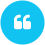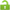ISSN

0975-6795 (Online)
2321-5828 (Print)

# Cost Function

Author(s):

Email(s): Email ID Not Available

DOI: Not Available

Hidayattullah National Law University, Raipur
*Corresponding Author:

ABSTRACT:
The cost function measures the minimum cost of producing a given level of output for some fixed factor prices. As such it summarizes information about the technological choices available to the firms. It turns out that the behavior of the cost function can tell us a lot about the nature of the firm's technology. The average total cost curve: The average total cost curve is constructed to capture the relation between average total cost and the level of output. A productively efficient firm organizes its factors of production in such a way that the average cost of production is at lowest point. A marginal cost Curve: A marginal cost Curve graphically represents the relation between marginal costs incurred by a firm and the quantity of output produced. This curve is constructed to capture the relation between marginal cost and the level of output, the marginal cost curve is U-shaped. Marginal cost is relatively high at small quantities of output, then as production increases, declines, reaches a minimum value, then rises. Duality: Given a cost function we can "solve for" a technology that could have generated that cost function. This means that the cost unction contains essentially the same information that the production function contains. Any concept defined in terms of the properties of the production function has a "dual" definition in terms of the properties of the cost function and vice versa. This general observation is known as the principle of duality.

Keywords:Nisha Nagwanshi. Cost Function. Research J. Humanities and Social Sciences. 3(2): April-June, 2012, 274-275.

## Recomonded Articles:

### Cost Function

Author(s): Nisha Nagwanshi,

DOI:         Access:Open Access Read MoreResearch Journal of Humanities and Social Sciences (RJHSS) is an international, peer-reviewed journal, correspondence in the fields of arts, commerce and social sciences....... Read more >>>

RNI: Not Available
DOI: 10.5958/2321-5828